# RS Aggarwal Solutions for Class 7 Maths Exercise 4D Chapter 4 Rational Numbers

RS Aggarwal Solutions for Class 7 Maths Exercise 4D Chapter 4 Rational Numbers in simple PDF are available here. The exercise of RS Aggarwal Solutions for Class 7 Chapter 4 contains the questions related to the subtraction of rational numbers.These exercises are formulated by our expert tutors in order to assist you with your exam preparation to attain good marks in Maths. Students who wish to score good marks in Maths practise RS Aggarwal Solutions for Class 7 Maths Chapter 4 Rational Numbers Exercise 4D.

## Download the PDF of RS Aggarwal Solutions For Class 7 Maths Chapter 4 Rational Numbers – Exercise 4D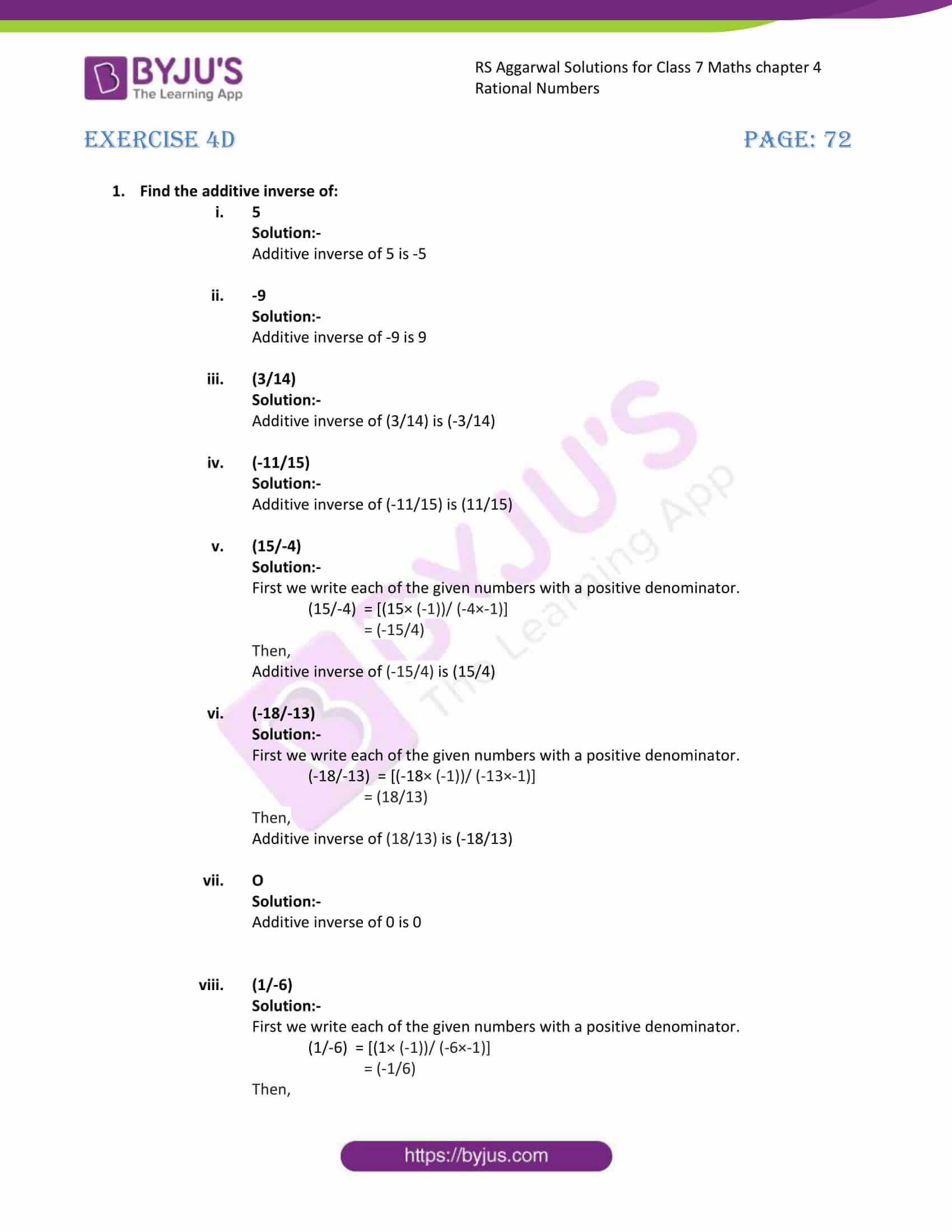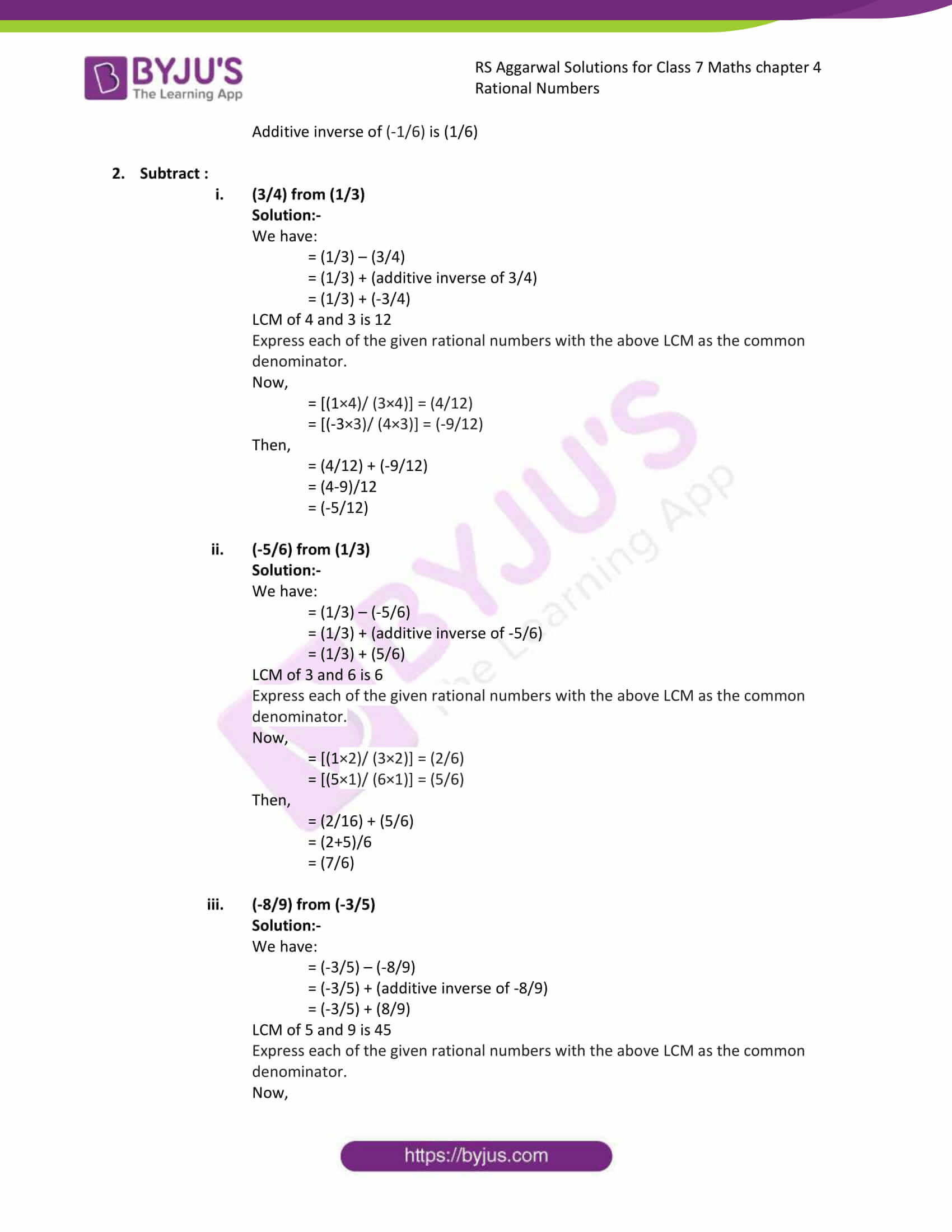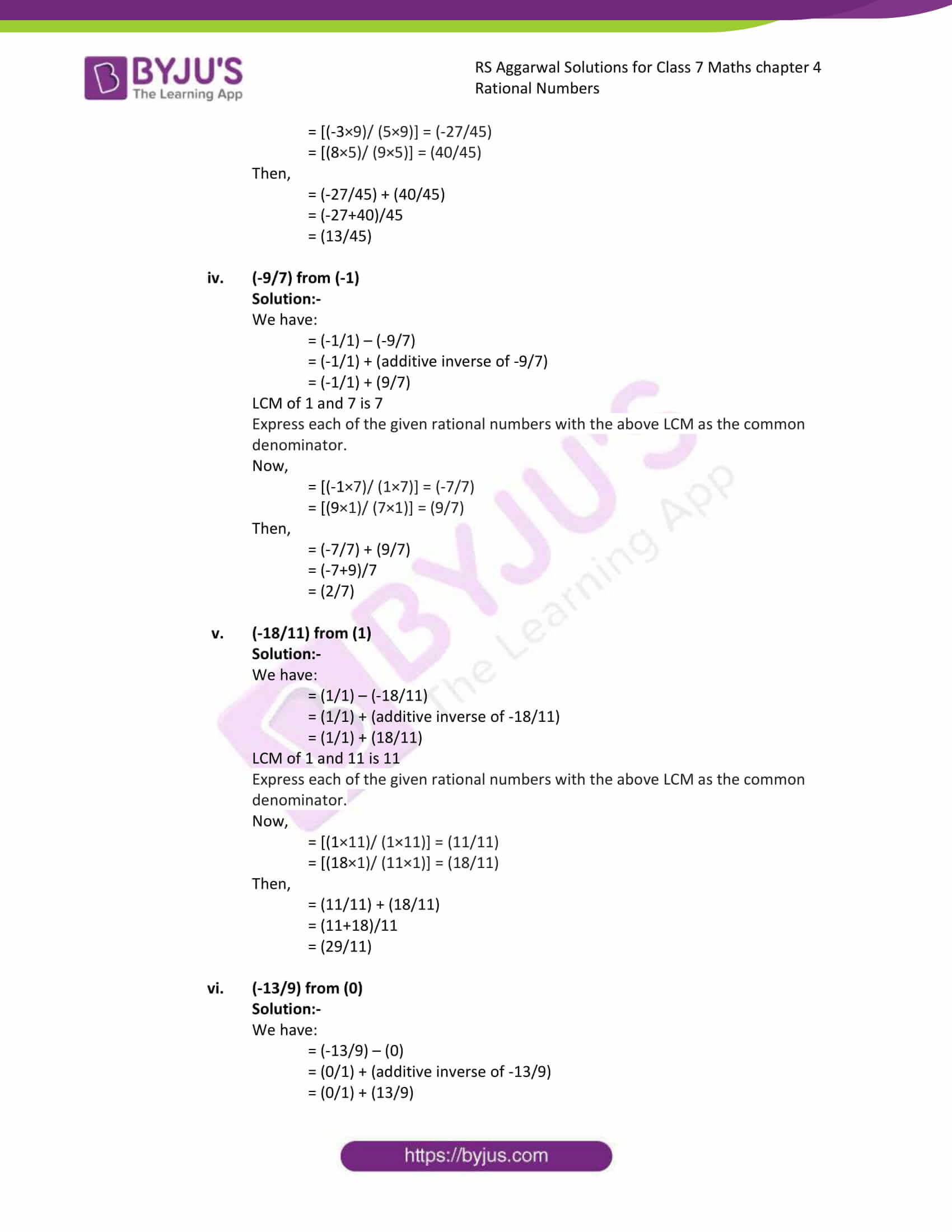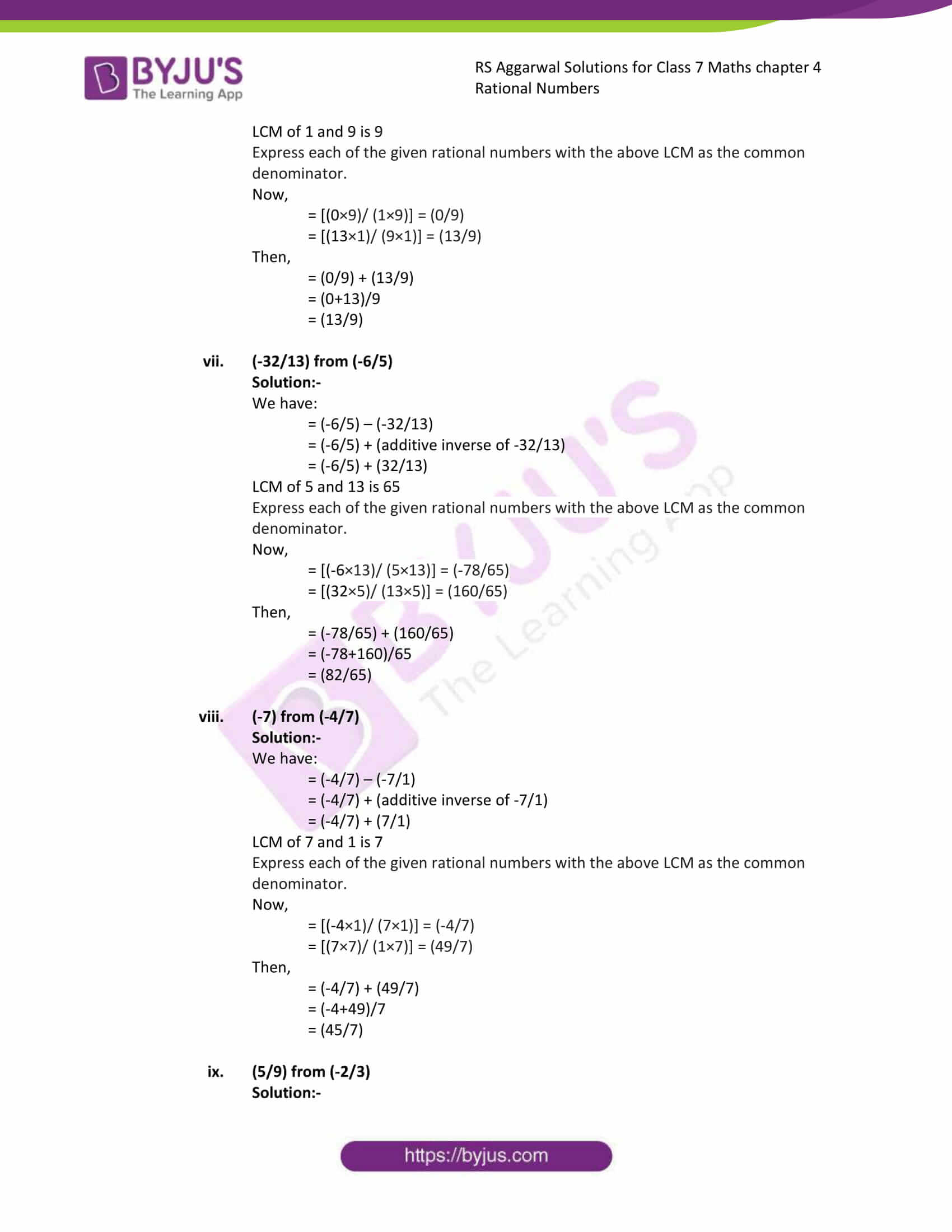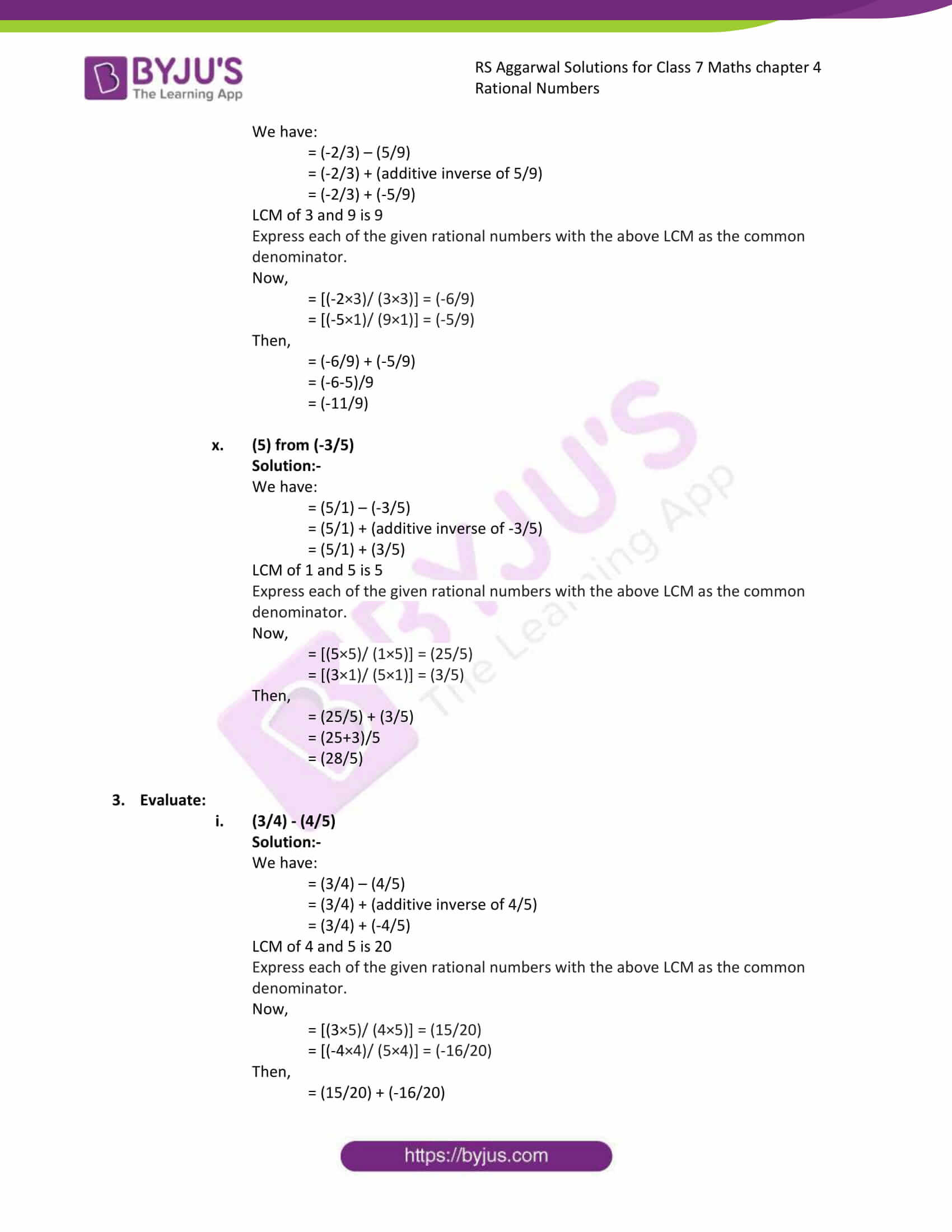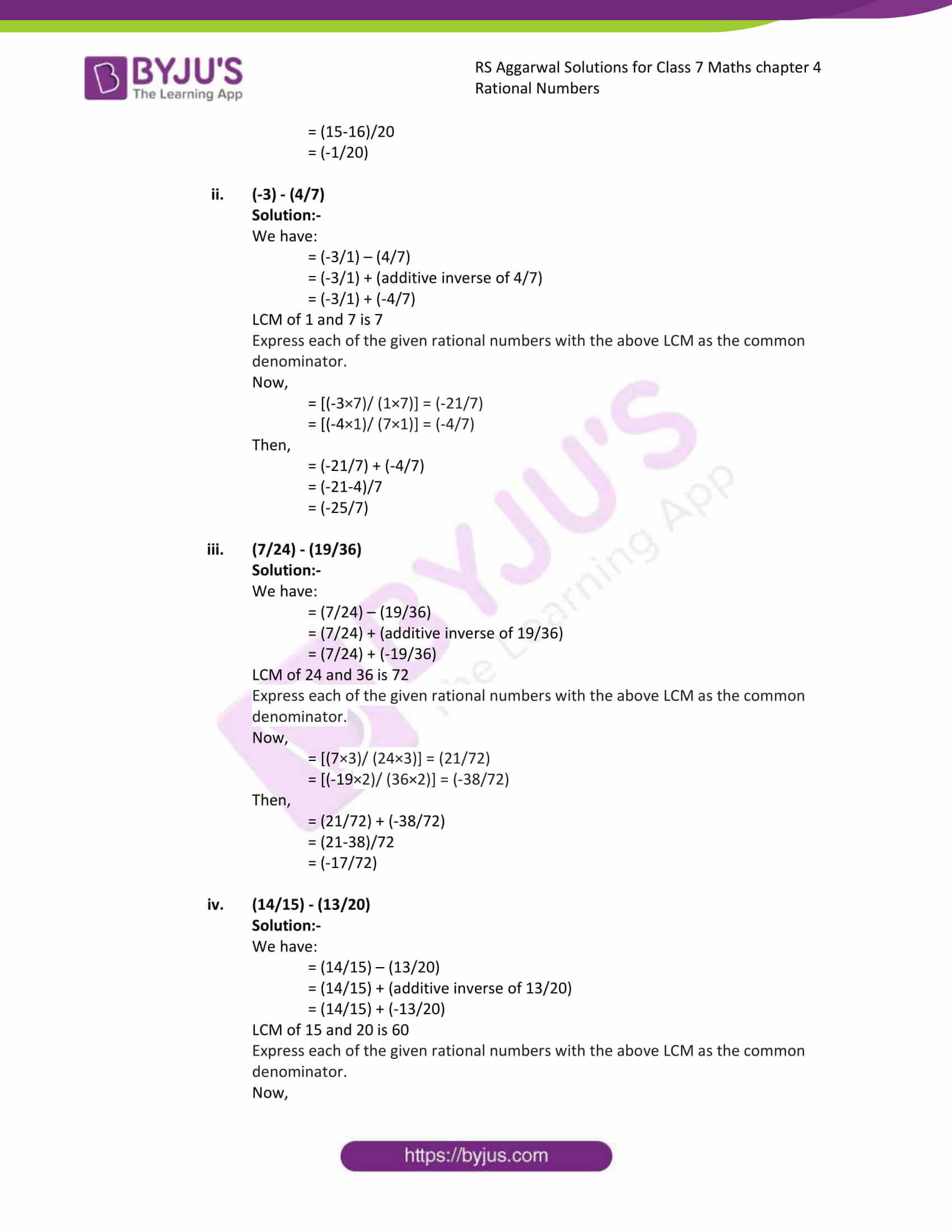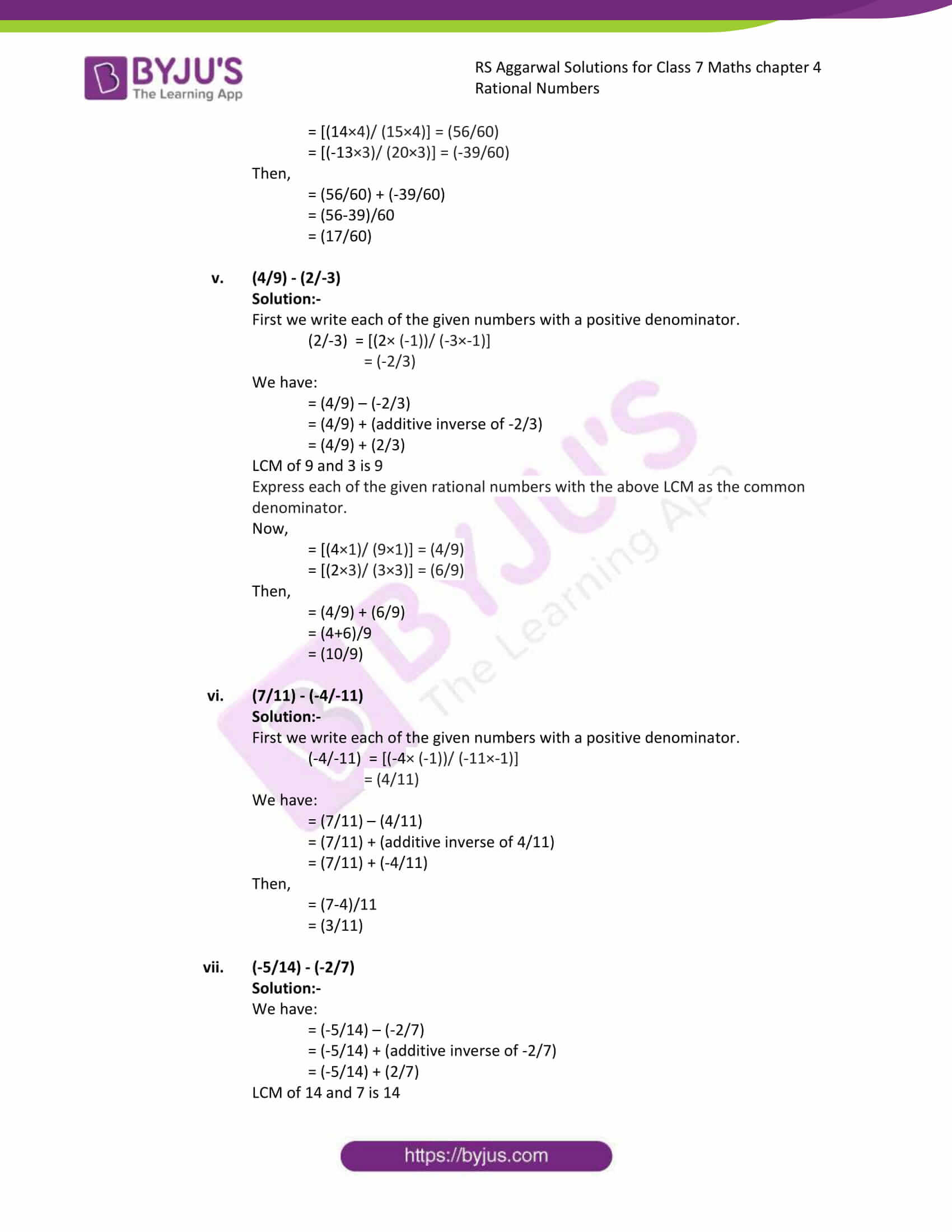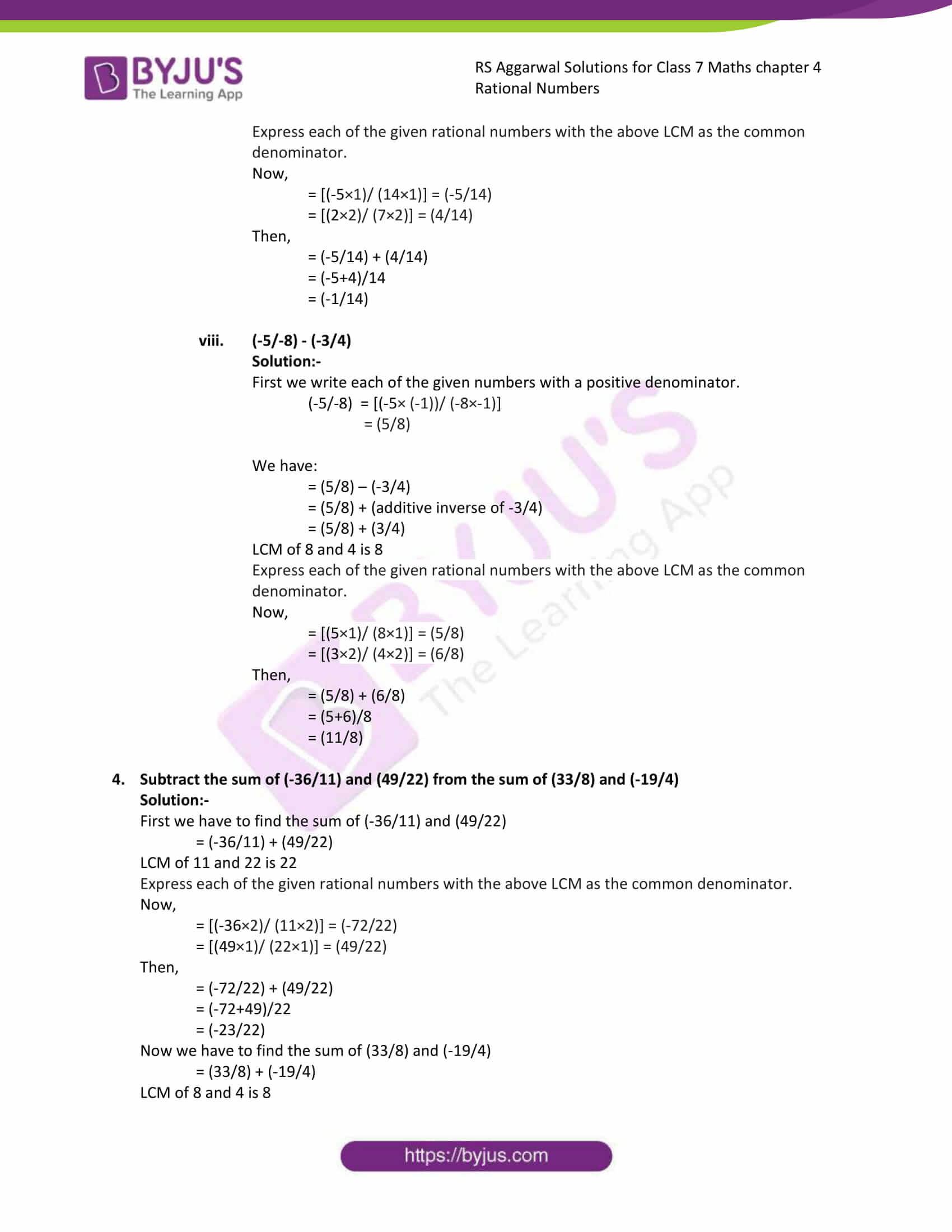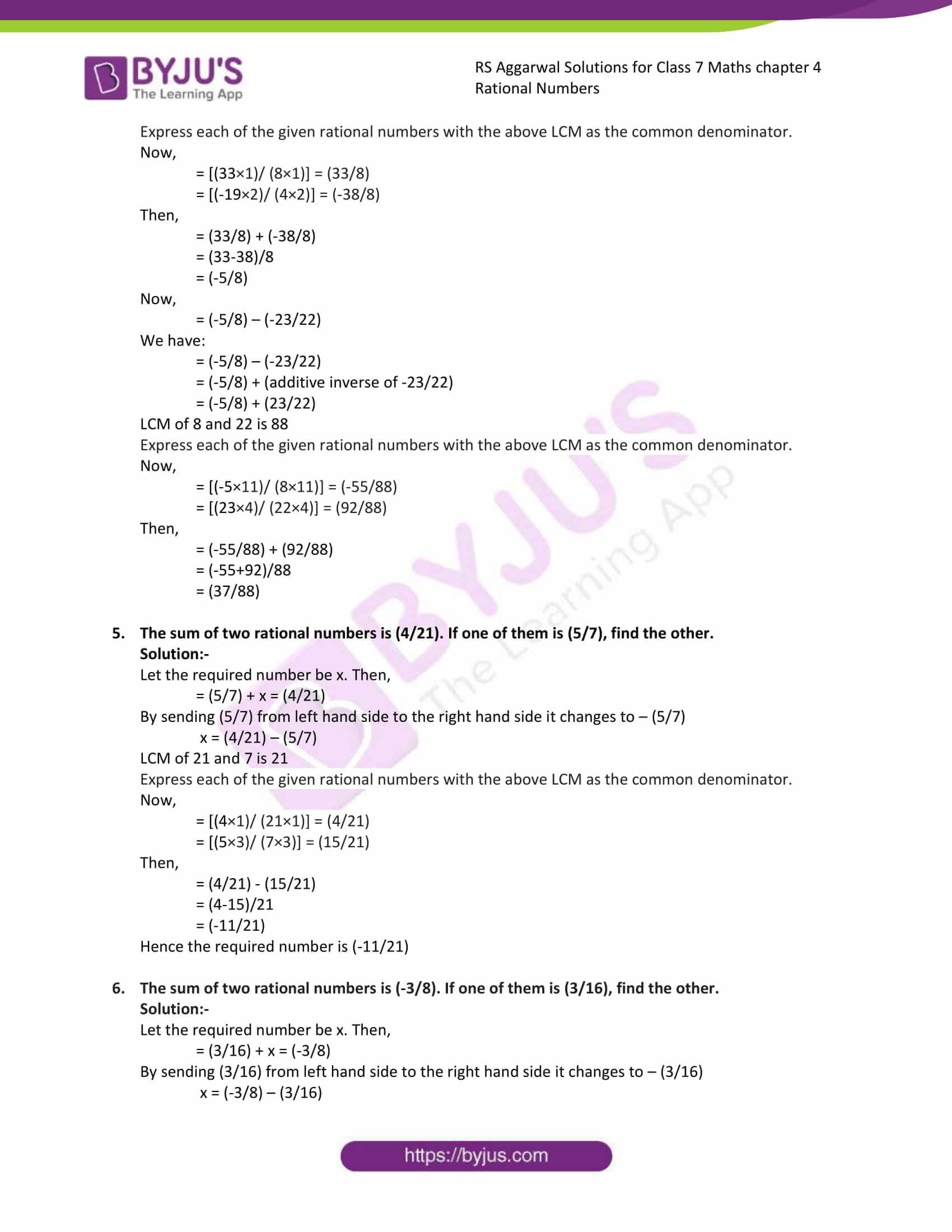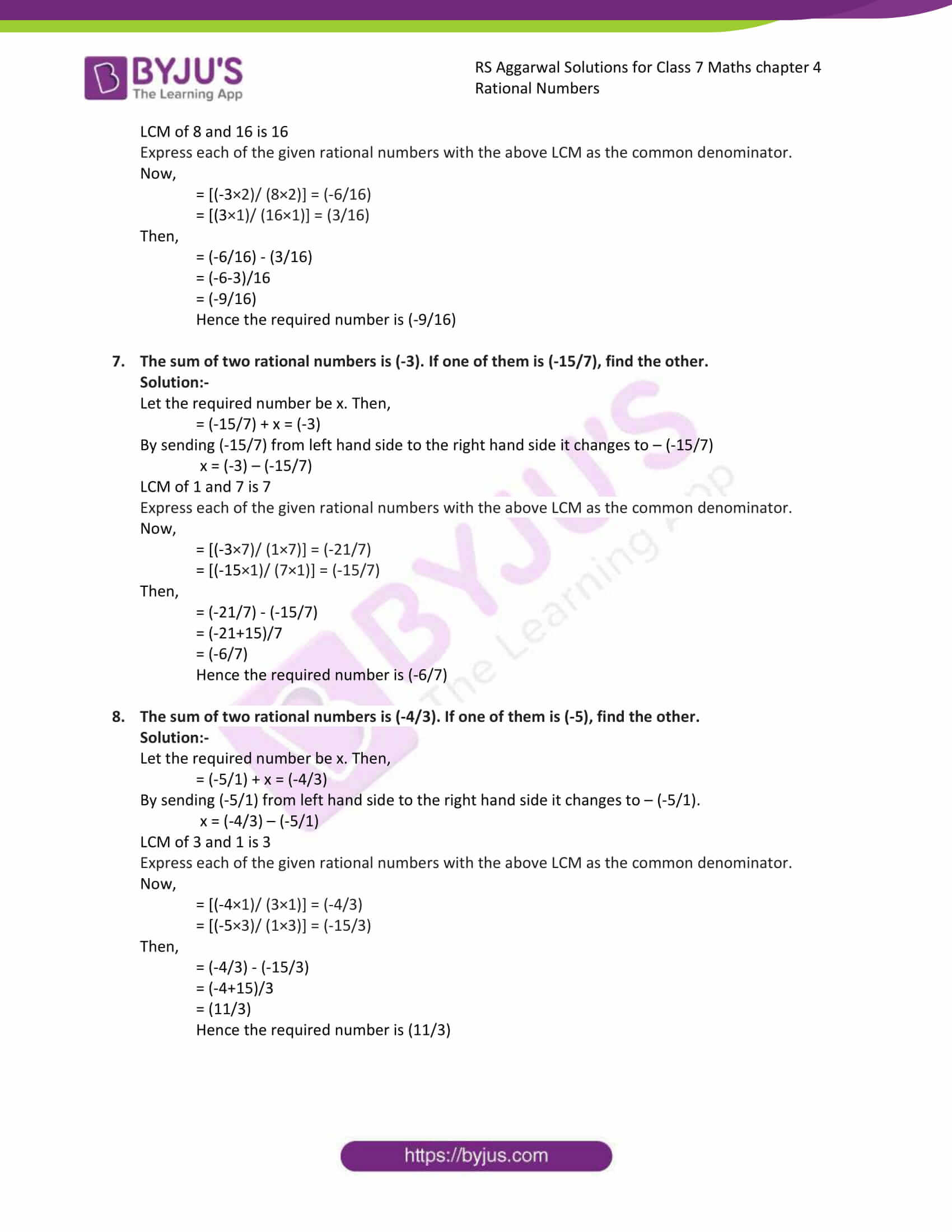### Access answers to Maths RS Aggarwal Solutions for Class 7 Chapter 4 – Rational Numbers Exercise 4D

1. Find the additive inverse of:
(i) 5

Solution:-

Additive inverse of 5 is -5

(ii) -9

Solution:-

Additive inverse of -9 is 9

(iii) (3/14)

Solution:-

Additive inverse of (3/14) is (-3/14)

(iv) (-11/15)

Solution:-

Additive inverse of (-11/15) is (11/15)

(v) (15/-4)

Solution:-

First we write each of the given numbers with a positive denominator.

(15/-4) = [(15× (-1))/ (-4×-1)]

= (-15/4)

Then,

Additive inverse of (-15/4) is (15/4)

(vi) (-18/-13)

Solution:-

First we write each of the given numbers with a positive denominator.

(-18/-13) = [(-18× (-1))/ (-13×-1)]

= (18/13)

Then,

Additive inverse of (18/13) is (-18/13)

(vii) O

Solution:-

Additive inverse of 0 is 0

(viii) (1/-6)

Solution:-

First we write each of the given numbers with a positive denominator.

(1/-6) = [(1× (-1))/ (-6×-1)]

= (-1/6)

Then,

Additive inverse of (-1/6) is (1/6)

2. Subtract :
(i) (3/4) from (1/3)

Solution:-

We have:

= (1/3) – (3/4)

= (1/3) + (additive inverse of 3/4)

= (1/3) + (-3/4)

LCM of 4 and 3 is 12

Express each of the given rational numbers with the above LCM as the common denominator.

Now,

= [(1×4)/ (3×4)] = (4/12)

= [(-3×3)/ (4×3)] = (-9/12)

Then,

= (4/12) + (-9/12)

= (4-9)/12

= (-5/12)

(ii) (-5/6) from (1/3)

Solution:-

We have:

= (1/3) – (-5/6)

= (1/3) + (additive inverse of -5/6)

= (1/3) + (5/6)

LCM of 3 and 6 is 6

Express each of the given rational numbers with the above LCM as the common denominator.

Now,

= [(1×2)/ (3×2)] = (2/6)

= [(5×1)/ (6×1)] = (5/6)

Then,

= (2/16) + (5/6)

= (2+5)/6

= (7/6)

(iii) (-8/9) from (-3/5)

Solution:-

We have:

= (-3/5) – (-8/9)

= (-3/5) + (additive inverse of -8/9)

= (-3/5) + (8/9)

LCM of 5 and 9 is 45

Express each of the given rational numbers with the above LCM as the common denominator.

Now,

= [(-3×9)/ (5×9)] = (-27/45)

= [(8×5)/ (9×5)] = (40/45)

Then,

= (-27/45) + (40/45)

= (-27+40)/45

= (13/45)

(iv) (-9/7) from (-1)

Solution:-

We have:

= (-1/1) – (-9/7)

= (-1/1) + (additive inverse of -9/7)

= (-1/1) + (9/7)

LCM of 1 and 7 is 7

Express each of the given rational numbers with the above LCM as the common denominator.

Now,

= [(-1×7)/ (1×7)] = (-7/7)

= [(9×1)/ (7×1)] = (9/7)

Then,

= (-7/7) + (9/7)

= (-7+9)/7

= (2/7)

(v) (-18/11) from (1)

Solution:-

We have:

= (1/1) – (-18/11)

= (1/1) + (additive inverse of -18/11)

= (1/1) + (18/11)

LCM of 1 and 11 is 11

Express each of the given rational numbers with the above LCM as the common denominator.

Now,

= [(1×11)/ (1×11)] = (11/11)

= [(18×1)/ (11×1)] = (18/11)

Then,

= (11/11) + (18/11)

= (11+18)/11

= (29/11)

(vi) (-13/9) from (0)

Solution:-

We have:

= (-13/9) – (0)

= (0/1) + (additive inverse of -13/9)

= (0/1) + (13/9)

LCM of 1 and 9 is 9

Express each of the given rational numbers with the above LCM as the common denominator.

Now,

= [(0×9)/ (1×9)] = (0/9)

= [(13×1)/ (9×1)] = (13/9)

Then,

= (0/9) + (13/9)

= (0+13)/9

= (13/9)

(vii) (-32/13) from (-6/5)

Solution:-

We have:

= (-6/5) – (-32/13)

= (-6/5) + (additive inverse of -32/13)

= (-6/5) + (32/13)

LCM of 5 and 13 is 65

Express each of the given rational numbers with the above LCM as the common denominator.

Now,

= [(-6×13)/ (5×13)] = (-78/65)

= [(32×5)/ (13×5)] = (160/65)

Then,

= (-78/65) + (160/65)

= (-78+160)/65

= (82/65)

(viii) (-7) from (-4/7)

Solution:-

We have:

= (-4/7) – (-7/1)

= (-4/7) + (additive inverse of -7/1)

= (-4/7) + (7/1)

LCM of 7 and 1 is 7

Express each of the given rational numbers with the above LCM as the common denominator.

Now,

= [(-4×1)/ (7×1)] = (-4/7)

= [(7×7)/ (1×7)] = (49/7)

Then,

= (-4/7) + (49/7)

= (-4+49)/7

= (45/7)

(ix) (5/9) from (-2/3)

Solution:-

We have:

= (-2/3) – (5/9)

= (-2/3) + (additive inverse of 5/9)

= (-2/3) + (-5/9)

LCM of 3 and 9 is 9

Express each of the given rational numbers with the above LCM as the common denominator.

Now,

= [(-2×3)/ (3×3)] = (-6/9)

= [(-5×1)/ (9×1)] = (-5/9)

Then,

= (-6/9) + (-5/9)

= (-6-5)/9

= (-11/9)

(x) (5) from (-3/5)

Solution:-

We have:

= (5/1) – (-3/5)

= (5/1) + (additive inverse of -3/5)

= (5/1) + (3/5)

LCM of 1 and 5 is 5

Express each of the given rational numbers with the above LCM as the common denominator.

Now,

= [(5×5)/ (1×5)] = (25/5)

= [(3×1)/ (5×1)] = (3/5)

Then,

= (25/5) + (3/5)

= (25+3)/5

= (28/5)

3. Evaluate:
(i) (3/4) – (4/5)

Solution:-

We have:

= (3/4) – (4/5)

= (3/4) + (additive inverse of 4/5)

= (3/4) + (-4/5)

LCM of 4 and 5 is 20

Express each of the given rational numbers with the above LCM as the common denominator.

Now,

= [(3×5)/ (4×5)] = (15/20)

= [(-4×4)/ (5×4)] = (-16/20)

Then,

= (15/20) + (-16/20)

= (15-16)/20

= (-1/20)

(ii) (-3) – (4/7)

Solution:-

We have:

= (-3/1) – (4/7)

= (-3/1) + (additive inverse of 4/7)

= (-3/1) + (-4/7)

LCM of 1 and 7 is 7

Express each of the given rational numbers with the above LCM as the common denominator.

Now,

= [(-3×7)/ (1×7)] = (-21/7)

= [(-4×1)/ (7×1)] = (-4/7)

Then,

= (-21/7) + (-4/7)

= (-21-4)/7

= (-25/7)

(iii) (7/24) – (19/36)

Solution:-

We have:

= (7/24) – (19/36)

= (7/24) + (additive inverse of 19/36)

= (7/24) + (-19/36)

LCM of 24 and 36 is 72

Express each of the given rational numbers with the above LCM as the common denominator.

Now,

= [(7×3)/ (24×3)] = (21/72)

= [(-19×2)/ (36×2)] = (-38/72)

Then,

= (21/72) + (-38/72)

= (21-38)/72

= (-17/72)

(iv) (14/15) – (13/20)

Solution:-

We have:

= (14/15) – (13/20)

= (14/15) + (additive inverse of 13/20)

= (14/15) + (-13/20)

LCM of 15 and 20 is 60

Express each of the given rational numbers with the above LCM as the common denominator.

Now,

= [(14×4)/ (15×4)] = (56/60)

= [(-13×3)/ (20×3)] = (-39/60)

Then,

= (56/60) + (-39/60)

= (56-39)/60

= (17/60)

(v) (4/9) – (2/-3)

Solution:-

First we write each of the given numbers with a positive denominator.

(2/-3) = [(2× (-1))/ (-3×-1)]

= (-2/3)

We have:

= (4/9) – (-2/3)

= (4/9) + (additive inverse of -2/3)

= (4/9) + (2/3)

LCM of 9 and 3 is 9

Express each of the given rational numbers with the above LCM as the common denominator.

Now,

= [(4×1)/ (9×1)] = (4/9)

= [(2×3)/ (3×3)] = (6/9)

Then,

= (4/9) + (6/9)

= (4+6)/9

= (10/9)

(vi) (7/11) – (-4/-11)

Solution:-

First we write each of the given numbers with a positive denominator.

(-4/-11) = [(-4× (-1))/ (-11×-1)]

= (4/11)

We have:

= (7/11) – (4/11)

= (7/11) + (additive inverse of 4/11)

= (7/11) + (-4/11)

Then,

= (7-4)/11

= (3/11)

(vii) (-5/14) – (-2/7)

Solution:-

We have:

= (-5/14) – (-2/7)

= (-5/14) + (additive inverse of -2/7)

= (-5/14) + (2/7)

LCM of 14 and 7 is 14

Express each of the given rational numbers with the above LCM as the common denominator.

Now,

= [(-5×1)/ (14×1)] = (-5/14)

= [(2×2)/ (7×2)] = (4/14)

Then,

= (-5/14) + (4/14)

= (-5+4)/14

= (-1/14)

(viii) (-5/-8) – (-3/4)

Solution:-

First we write each of the given numbers with a positive denominator.

(-5/-8) = [(-5× (-1))/ (-8×-1)]

= (5/8)

We have:

= (5/8) – (-3/4)

= (5/8) + (additive inverse of -3/4)

= (5/8) + (3/4)

LCM of 8 and 4 is 8

Express each of the given rational numbers with the above LCM as the common denominator.

Now,

= [(5×1)/ (8×1)] = (5/8)

= [(3×2)/ (4×2)] = (6/8)

Then,

= (5/8) + (6/8)

= (5+6)/8

= (11/8)

4. Subtract the sum of (-36/11) and (49/22) from the sum of (33/8) and (-19/4)

Solution:-

First we have to find the sum of (-36/11) and (49/22)

= (-36/11) + (49/22)

LCM of 11 and 22 is 22

Express each of the given rational numbers with the above LCM as the common denominator.

Now,

= [(-36×2)/ (11×2)] = (-72/22)

= [(49×1)/ (22×1)] = (49/22)

Then,

= (-72/22) + (49/22)

= (-72+49)/22

= (-23/22)

Now we have to find the sum of (33/8) and (-19/4)

= (33/8) + (-19/4)

LCM of 8 and 4 is 8

Express each of the given rational numbers with the above LCM as the common denominator.

Now,

= [(33×1)/ (8×1)] = (33/8)

= [(-19×2)/ (4×2)] = (-38/8)

Then,

= (33/8) + (-38/8)

= (33-38)/8

= (-5/8)

Now,

= (-5/8) – (-23/22)

We have:

= (-5/8) – (-23/22)

= (-5/8) + (additive inverse of -23/22)

= (-5/8) + (23/22)

LCM of 8 and 22 is 88

Express each of the given rational numbers with the above LCM as the common denominator.

Now,

= [(-5×11)/ (8×11)] = (-55/88)

= [(23×4)/ (22×4)] = (92/88)

Then,

= (-55/88) + (92/88)

= (-55+92)/88

= (37/88)

5. The sum of two rational numbers is (4/21). If one of them is (5/7), find the other.

Solution:-

Let the required number be x. Then,

= (5/7) + x = (4/21)

By sending (5/7) from left hand side to the right hand side it changes to – (5/7)

x = (4/21) – (5/7)

LCM of 21 and 7 is 21

Express each of the given rational numbers with the above LCM as the common denominator.

Now,

= [(4×1)/ (21×1)] = (4/21)

= [(5×3)/ (7×3)] = (15/21)

Then,

= (4/21) – (15/21)

= (4-15)/21

= (-11/21)

Hence the required number is (-11/21)

6. The sum of two rational numbers is (-3/8). If one of them is (3/16), find the other.

Solution:-

Let the required number be x. Then,

= (3/16) + x = (-3/8)

By sending (3/16) from left hand side to the right hand side it changes to – (3/16)

x = (-3/8) – (3/16)

LCM of 8 and 16 is 16

Express each of the given rational numbers with the above LCM as the common denominator.

Now,

= [(-3×2)/ (8×2)] = (-6/16)

= [(3×1)/ (16×1)] = (3/16)

Then,

= (-6/16) – (3/16)

= (-6-3)/16

= (-9/16)

Hence the required number is (-9/16)

7. The sum of two rational numbers is (-3). If one of them is (-15/7), find the other.

Solution:-

Let the required number be x. Then,

= (-15/7) + x = (-3)

By sending (-15/7) from left hand side to the right hand side it changes to – (-15/7)

x = (-3) – (-15/7)

LCM of 1 and 7 is 7

Express each of the given rational numbers with the above LCM as the common denominator.

Now,

= [(-3×7)/ (1×7)] = (-21/7)

= [(-15×1)/ (7×1)] = (-15/7)

Then,

= (-21/7) – (-15/7)

= (-21+15)/7

= (-6/7)

Hence the required number is (-6/7)

8. The sum of two rational numbers is (-4/3). If one of them is (-5), find the other.

Solution:-

Let the required number be x. Then,

= (-5/1) + x = (-4/3)

By sending (-5/1) from left hand side to the right hand side it changes to – (-5/1).

x = (-4/3) – (-5/1)

LCM of 3 and 1 is 3

Express each of the given rational numbers with the above LCM as the common denominator.

Now,

= [(-4×1)/ (3×1)] = (-4/3)

= [(-5×3)/ (1×3)] = (-15/3)

Then,

= (-4/3) – (-15/3)

= (-4+15)/3

= (11/3)

Hence the required number is (11/3)

### Access other exercises of RS Aggarwal Solutions For Class 7 Chapter 4 – Rational Numbers

Exercise 4G Solutions